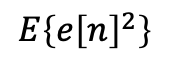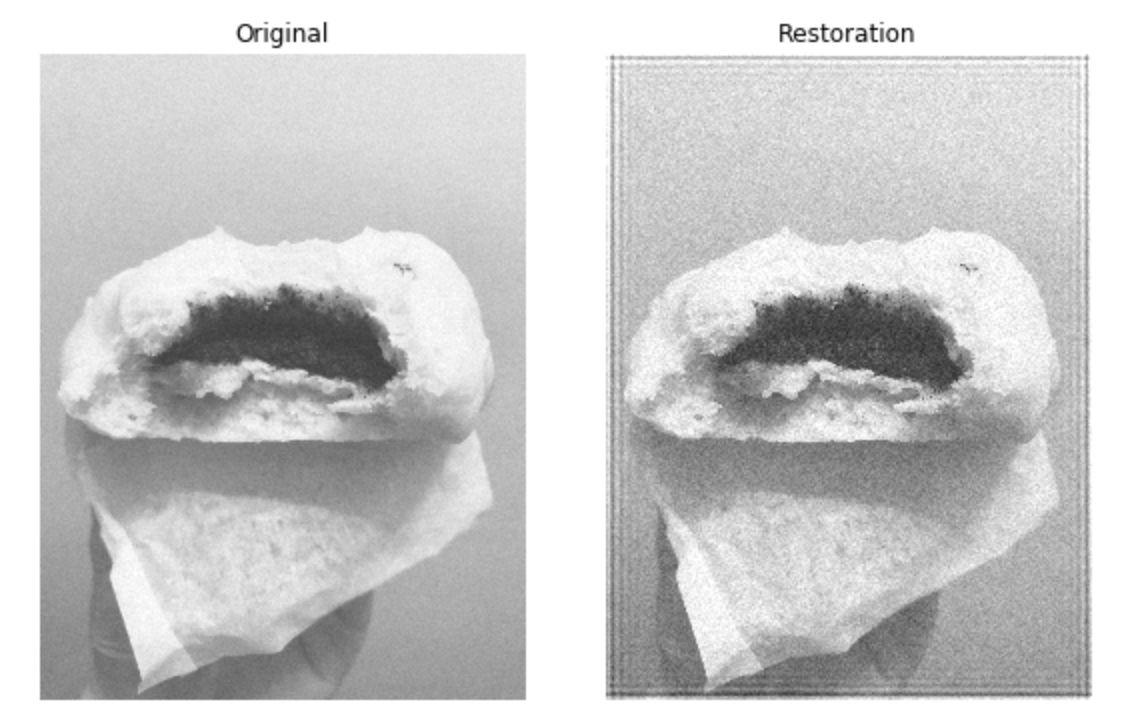×

Search anything:

# Wiener filter

#### Machine Learning (ML)We've taken some instagram worthy image - except that it was blurred and now, we want to get back the original image, or at least an approximation of it. How does that work?

# Background information

## What is the Wiener filter?

The Wiener filter performs two main functions - it inverts the blur of the image and removes extra noise. It is particularly helpful when processing images that have been through a degradation filter or when the image has been blurred by a known lowpass filter. It is often used in deconvolution, which is an algorithm-based process to enhance signals from data.

## How does the Wiener filter work?

The filter is based on a statistical approach.

It works according to an equation similar to the least squares method and the main purpose is to minimise the overall mean square error, or the average squared distance between the filtered output and a desired signal - the difference between the original and output signal should be minimised.

It assumes that the user knows the properties of the original signal and noise, such as the power functions for both the original signal and noise. It also requires the signal and noise to be linear stochastic processes with known spectral properties.

The Wiener filter can be subdivided into three problems, filtering, smoothing and prediction. d[n] represents the predicted signal, s[n] is the current signal and [k] is the change in signal, where k > 0. Filtering means estimating the current signal, that is d[n] = s[n]; smoothing is estimating past signal values so d[n] = s[n-k]; prediction is estimating future signal values so d[n] = s[n+k].

Consider a Wiener filter in the frequency domain W(u,v). The restored image should be X(u,v) = W(u,v).Y(u,v), where X is the restored output image and Y is the input signal.

## How do we derive the Wiener filter?

Define the initial input image to be x[n] which is equal to them sum of s[n], the zero mean, and w[n], the wide sense stationary process.

i.e. x[n] = s[n] + w[n]

The objective of the Wiener filter is to pass the input image H(z) through the filter H(z), which is to be chosen, so e[n], the error or the difference between the estimated and output filter, is as small as possible.The method for minimisation, or rather the cost function, is chosen to be by using the mean square error, so we try to minimise the below.This yields a parabolic curve of which we want to find the minimum point.First, we need to find a linear estimator, or a filter for d[n]Substituting this into the minimisation of mean square error equation, and with a vector h containing the impulse response coefficients h[k], we get the followingThese give us a linear minimum mean square estimator for the Wiener filter (d^)[n]

To solve the equation, first we consider that the function is quadratic in the vector h, as shown in the diagram of the mean square error. It is convex and has no local optima, so we can differentiate and set the derivative to zero. By using partial differentiation, we get the Wiener-Hopt (W-H) equations, as follows:for all t where h(t) is free to select

## Why use the Wiener filter?

Another option we could use would be inverse filtering, which is very sensitive to additive noise. The Wiener filter is the most common type of deconvolution filter used because it is the most mathematically correct one.

# The story behind the Wiener filter

This was a filter developed by Norbert Wiener in 1940 and published in 1949. It's main goal is noise reduction in a signal, and the filter does so by comparing the received signal with an estimation of the noiseless signal. This filter assumes the input to be stationery so it is not an adaptive filter.

# Code Implementation

First, let's import the necessary libraries

``````import skimage
from skimage.viewer import ImageViewer
from skimage import color, data, restoration
import sys
import numpy as np
import matplotlib.pyplot as plt
``````

Next, we import an image that is not blurred. Here we are importing a photo of a Chinese steamed bun (also called bao, but here we will use the variable name bun). Then we check that the image is correct by calling ImageViewer.

``````image = skimage.io.imread(fname="noblur.jpg")
viewer = ImageViewer(image)
``````

Then we convert the image to grayscale and add in a convolution. We can import convolve2d from scipy.signal which takes five arguments, in1, in2, mode, boundary, fillvalue. It will convolve in1 and in2, which should be of the same size and the output size will be determined by mode. Here we use the mode 'same' because we want the convolved image to be the same size as our original bun image, and centered with respect to the ‘full’ output. The boundary conditions are determined by boundary and fillvalue, which we have not used here - to read more, the documentation is available here.

Here in fact we are implementing the data model "y = Hx + n". On the left hand side, y is the image we have to deconvolve. On the right hand side, we have H which is the psf and x which is the original unknown image, as well as n, the noise.

``````bun = color.rgb2gray(image)
from scipy.signal import convolve2d
psf = np.ones((10, 10)) / 100
bun = convolve2d(bun, psf, 'same')
bun += 0.1 * bun.std() * np.random.standard_normal(bun.shape)
``````

Next, we work out the deconvolved image with

``````deconvolved, _ = restoration.unsupervised_wiener(bun, psf)
``````

We then plot this figure with matplotlib shown as follows.

``````fig, ax = plt.subplots(nrows=1, ncols=2, figsize=(8, 5),
sharex=True, sharey=True)

plt.gray()

ax.imshow(bun, vmin=deconvolved.min(), vmax=deconvolved.max())
ax.axis('off')
ax.set_title('Original bun')

ax.imshow(deconvolved)
ax.axis('off')
ax.set_title('Restored bun')

fig.tight_layout()

plt.show()
``````

After running the code, we get the following image - the original image has been restored quite well.It's rather grainy but that's because of the amount of noise we put in the original photo - by lowering the value of the parameters of the psf, we can obtain a "better restored" image.

# Application and Conclusion

Wiener filters play a central role in a wide range of applications such as linear prediction, echo cancellation, signal restoration, channel equalisation and system identification. This is a fairly expensive filter, in terms of computational cost and time, it is applied on every pixel of the image. As we have seen in this code example, the filter is fairly good at deblurring images and reducing noise.#### Annie Lee

Economics and Finance | Intern at OpenGenus